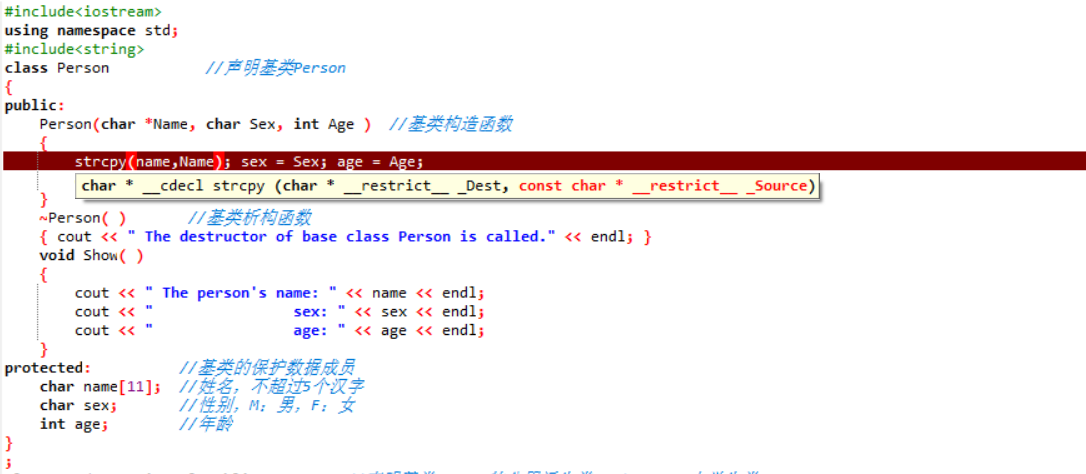2020-06-03 10:21

# 请问大佬们这个图里面为什么会报错啊？（下面是全部完整的代码）
#include<iostream
using namespace std;
#include<string

class Person //声明基类Person

{

public:

`````` Person(char *Name, char Sex, int Age )  //基类构造函数
{
strcpy(name, Name); sex = Sex; age = Age;
cout << " The constructor of base class Person is called." << endl;
}
~Person( )       //基类析构函数
{ cout << " The destructor of base class Person is called." << endl; }
void Show( )
{
cout << " The person's name: " << name << endl;
cout << "                sex: " << sex << endl;
cout << "                age: " << age << endl;
}
``````

protected: //基类的保护数据成员

``````    char name;  //姓名，不超过5个汉字
char sex;       //性别，M：男，F：女
int age;        //年龄
``````

}
;

class Student: virtual public Person //声明基类Person的公用派生类Student——大学生类

{

public:

``````    Student(char *Name, char Sex, int Age, char *Id, char *Date, float Score): Person(Name, Sex, Age) //派生类构造函数
{   //在构造函数的函数体中只对派生类新增的数据成员初始化
strcpy(id, Id); strcpy(date, Date); score = Score;
cout << " The constructor of derived class Student is called." << endl;
}
~Student( )       //派生类析构函数
{ cout << " The destructor of derived class Student is called." << endl; }
void StuShow( )
{
cout << "       student's id: " << id << endl;
cout << "               name: " << name << endl;
cout << "                sex: " << sex << endl;
cout << "                age: " << age << endl;
cout << "    enrollment date: " << date << endl;
cout << "   enrollment score: " << score << endl;
}
``````

protected:

`````` char id;     //学号，固定为11位

char date;   //入学时间

float score;     //入学成绩
``````

};

{

public:
//派生类的构造函数

``````    Graduate(char *Name, char Sex, int Age, char *Id, char *Date, float Score, char *Direct, char *Teacher)
:Person(Name, Sex, Age),Student(Name, Sex, Age, Id, Date, Score)
{   //在构造函数的函数体中只对派生类所新增的数据成员初始化
strcpy(direct, Direct); strcpy(teacher, Teacher);
cout << " The constructor of derived class Graduate is called." << endl;
}
{
StuShow( );
cout << "             direct: " << direct << endl;
cout << "       teacher name: " << teacher << endl;
}
{  cout << " The destructor of derived class Graduate is called." << endl; }
``````

protected: //派生类的保护数据成员

`````` char direct;     //研究方向，不超过10个汉字
char teacher;    //导师姓名，不超过5个汉字
``````

};

class Employee:

virtual public Person //声明基类Person的公用派生类Employee——职工类

{

public:

``````    Employee(char *Name, char Sex, int Age, char *Num, char *Clerk, char *Depart, char *Timer): Person(Name, Sex, Age) //派生类构造函数
{
strcpy(num, Num);  strcpy(clerk, Clerk);
strcpy(department, Depart);  strcpy(timer, Timer);
cout << " The constructor of derived class Employee is called."<< endl;
}
~ Employee( )       //派生类析构函数
{  cout << " The destructor of derived class Employee is called." << endl; }
void EShow( )
{
cout << "    employee’s num: " << num << endl;
cout << "              clerk: " << clerk << endl;
cout << "         department: " << department << endl;
cout << "              timer: " << timer << endl;
}
void EmpShow( )
{
Show( );
cout << "    employee’s num: " << num << endl;
cout << "              clerk: " << clerk << endl;
cout << "         department: " << department << endl;
cout << "              timer: " << timer << endl;
}
``````

protected: //派生类的保护数据成员

``````    char num;         //职工编号，固定为7位字符
char clerk;        //职称，不超过10个汉字
char department;  //部门，不超过15个汉字
char timer;       //工作时间
``````

};

{

public:

``````    GradOnWork(char *Name, char Sex, int Age, char *Id, char *Date, float Score,
char *Direct, char *Teacher, char *Num, char *Clerk, char *Depart, char *Timer):
Person(Name, Sex, Age),
Graduate(Name, Sex, Age, Id, Date, Score, Direct, Teacher),
Employee(Name, Sex, Age, Num, Clerk, Depart, Timer) //派生类构造函数
{
cout << " The constructor of derived class GradOnWork is called."<< endl;
}
{
cout << " The destructor of derived class GradOnWork is called." << endl;
}
void GWShow( )
{
cout << "    Be the graduate:" << endl;
cout << "    Be the employee:" << endl;
EShow( );
}
``````

};

int main( )

{

``````GradOnWork obj("Mary", 'F', 19, "20120101001", "2012.09.01", 680,
"Computer", "Johnson", "JG01029", "Senior Engineer", "Research Department", "20 years");
obj.Show( );//只输出在职研究生的姓名、性别和年龄
return 0;
``````

}

• 写回答
• 关注问题
• 收藏
• 邀请回答